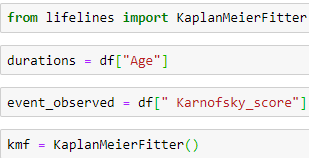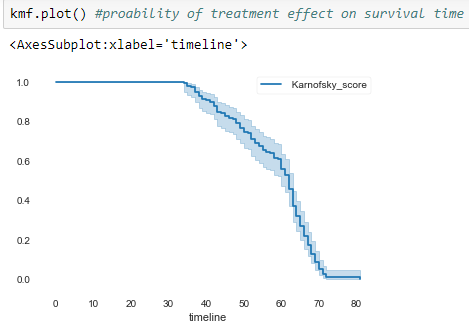Debyendu Dey — December 9, 2020

This article was published as a part of the Data Science Blogathon.

## Introduction

Survival Analysis

Many of us always have a question in the mind as to how much time will take for an event to happen. Like the failure of a mechanical system, the human body getting affected by any kind of disease, how much time it will take to cure the diseases. Then how many will survive a specific after doing a medical diagnosis, at what rate one will die or fail? Is it possible to take into consideration the multiple causes of death or failure be taken into the broader view? To answer all these questions we study Survival Analysis.

Survival Analysis is an important branch of Statistics that is taken into consideration to answer all these questions.

Survival Analysis study needs to define a time frame in which this study is carried out. As in many cases, it is possible that the given time-period for the event to occur is the same as each other. Survival analysis involves the modeling of time to event data. So, we need to define the context of Survival Analysis in the study like time as the “Event” in the context of Survival analysis.

There are different ways in which we perform survival analysis. It is performed in several ways like when we define a group. Some of them are Kaplan Meier Curves, Cox Regression Models, Hazard Function, Survival Function, etc.

When the Survival Analysis is done to compare the survival analysis of two different groups. There we perform the Log-Rank test.

When the Survival Analysis like to describe the categorical and quantitative variables on survival we like to do Cox proportional hazards regression, Parametric Survival Models, etc.

In the Survival Analysis, we need to define certain terms before one proceeds like the Event, Time, Censoring, Survival Function, etc.

Event, when we talk about, is the activity which is going on or is going to happen in the survival analysis study like the Death of a Person from a particular disease, time to get cure by a medical diagnose, time to get cured by vaccines, time of occurrence of failure of machines in the manufacturing shop floor, time for diseases occurrence, etc.

#### Time

in survival analysis case study is the time from the beginning of the survival analysis observation on the subject matter till the time when the event is going to occur. Like in the case of Mechanical Machine to a failure we need to know the

(a) time of an event when the machine will start
(b) when the machine will fail
(c) loss of machine or the shutdown of the machine from the survival analysis study.

## Censoring/ Censored Observation

This terminology is defined as if the subject matter on which we are doing the study of survival analysis doesn’t get affected by the defined event of study, then they are described as censored. The censored subject might also not have an event after the end of the survival analysis observation. The subject is called censored in the sense that nothing was observed out of the subject after the time of censoring.

Censoring Observation are also of 3 types-

#### 1. Right Censored

Right censoring is used in many problems. It happens when we are not certain what happened to people after a certain point in time.

It occurs when the true event time is greater than the censored time when c < t. This happens if either some people cannot be followed the entire time because they died or were lost to follow up or withdrew from the study.

#### 2. Left Censored

Left censoring is when we are not certain what happened to people before some point in time. Left censoring is the opposite, occurring when the true event time is less than the censored time when c > t.

#### 3. Interval Censored

Interval censoring is when we know something has happened in an interval (not before starting time and not after ending time of the study) but we do not know exactly when in the interval it happened.

Interval censoring is a concatenation of the left and right censoring when the time is known to have occurred between two-time points

Survival Function S (t): This is a probability function that depends on the time of the study. The subject survives more than time t. The Survivor function gives the probability that the random variable T exceeds the specified time t.

Here, we will discuss the Kaplan Meier Estimator.

## Kaplan Meier Estimator

Kaplan Meier Estimator is used to estimate the survival function for lifetime data. It is a non-parametric statistics technique. It is also known as the product-limit estimator, and the concept lies in estimating the survival time for a certain time of like a major medical trial event, a certain time of death, failure of the machine, or any major significant event.

There are lots of examples like

1. Failure of machine parts after several hours of operation.

2. How much time it will take for COVID 19 vaccine to cure the patient.

3. How much time is required to get a cure from a medical diagnosis etc.

4. To estimate how many employees will leave the company in a specific period of time.

5. How many patients will get cured by lung cancer

To Estimate the Kaplan Meier Survival we first need to estimate the Survival Function S (t) is the probability of event time t

Where (d) are the number of death events at the time (t), and (n) is the number of subjects at risk of death just prior to the time (t).

## Assumptions of Kaplan Meier Survival

In real-life cases, we do not have an idea of the true survival rate function. So in Kaplan Meier Estimator we estimate and approximate the true survival function from the study data. There are 3 assumptions of Kaplan Meier Survival

1) Survival Probabilities are the same for all the samples who joined late in the study and those who have joined early. The Survival analysis which can affect is not assumed to change.

2) Occurrence of Event are done at a specified time.

3) Censoring of the study does not depend on the outcome. The Kaplan Meier method doesn’t depend on the outcome of interest.

Interpretation of Survival Analysis is Y-axis shows the probability of subject which has not come under the case study. The X-axis shows the representation of the subject’s interest after surviving up to time. Each drop in the survival function (approximated by the Kaplan-Meier estimator) is caused by the event of interest happening for at least one observation.

The plot is often accompanied by confidence intervals, to describe the uncertainty about the point estimates-wider confidence intervals show high uncertainty, this happens when we have a few participants- occurs in both observations dying and being censored.

## Important things to consider for Kaplan Meier Estimator Analysis

1) We need to perform the Log Rank Test to make any kind of inferences.

2) Kaplan Meier’s results can be easily biased. The Kaplan Meier is a univariate approach to solving the problem

3) Removal of Censored Data will cause to change in the shape of the curve. This will create biases in model fit-up

4) Statistical tests and observations become mislead if the Dichotomizing of Continuous Variable is performed.

5) By dichotomizing means we take statistical measures such as median to create groups but this may lead to problems in the data set.

## Let us take the example in Python

Let us import the important library required to work in python

First, we are importing different python libraries for our work. Here, we are taking the lung-cancer data set. After the libraries and load, we will read the data using the pandas library. The data set contains different information

Treatment 1=standard, 2=test, Cell type 1=squamous, 2=small
cell, 3=adeno, 4=large, Survival in days, Status 1=dead, 0=censored, Karnofsky score (a measure of general performance, 100=best), Months from Diagnosis, Age in years Prior therapy 0=no, 10=yes, etc.

Here we see the Head &tail.Now, Here we import the python code for performing the Kaplan Meier EstimatorHere, we perform the analysis on the Karnofsky score it x-axis depicts the timeline and the y-axis shows the score. The best score is 1 it means the subject is fit, a score of 0 means the worst score.

Then we apply the code for Survival, Prior Therapy, the treatment here we will do the Kaplan Meier Estimator Analysis.

Then, we fit up kmf1 = KaplanMeierFitter() for fitting up the Kaplan Meier function and we run the following code for different data related to the lung cancer problems.

Kaplan Meier estimator after running the code shows the plot between Treatment test standard &Treatment test.

In this paper, my key objective was to explain the Survival Analysis with Kaplan Meier Estimator. The things related to it and a problem description in real life.

1) Does not require too many features- time to the survival analysis event is only required.

2) Provides an average overview related to the event.